Rotational Symmetry

Chapter 12 Class 7 Symmetry
Concept wise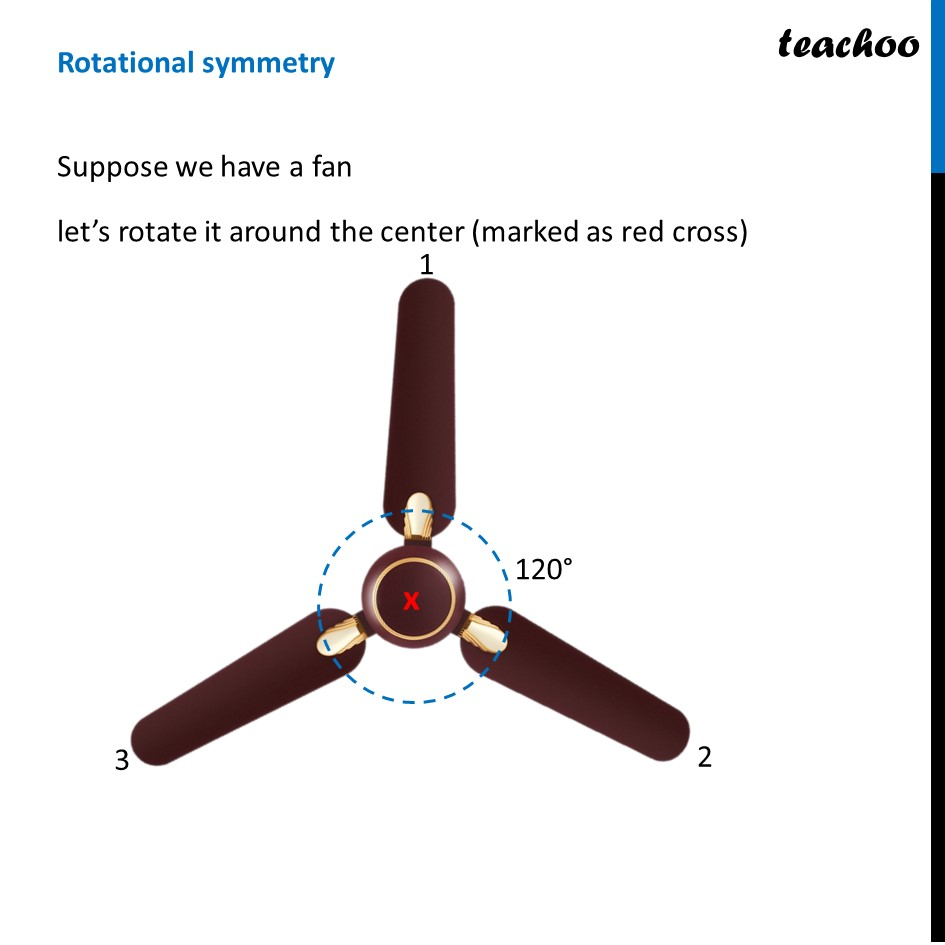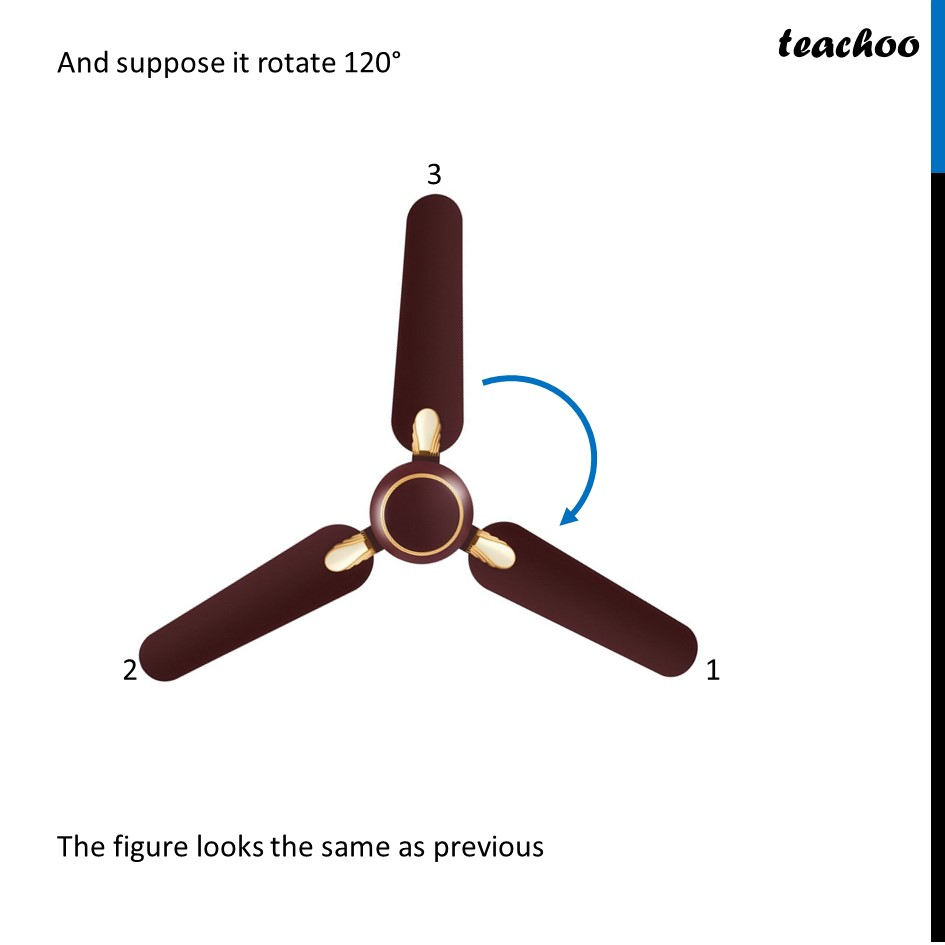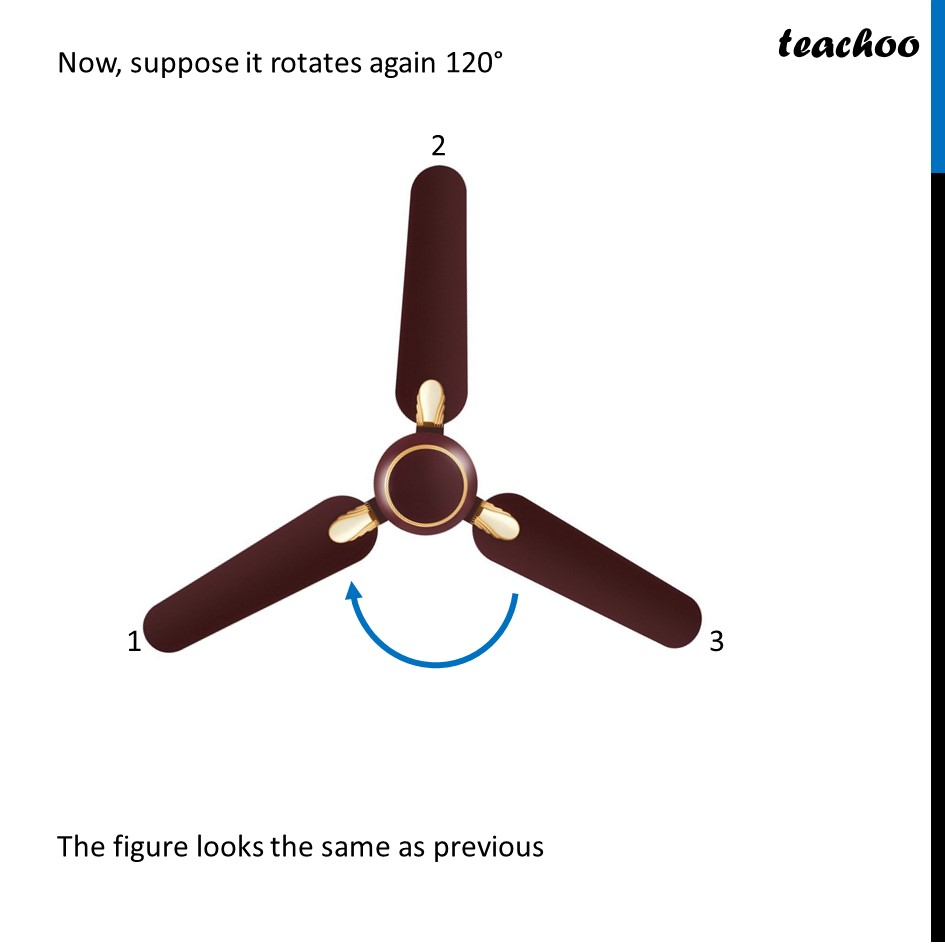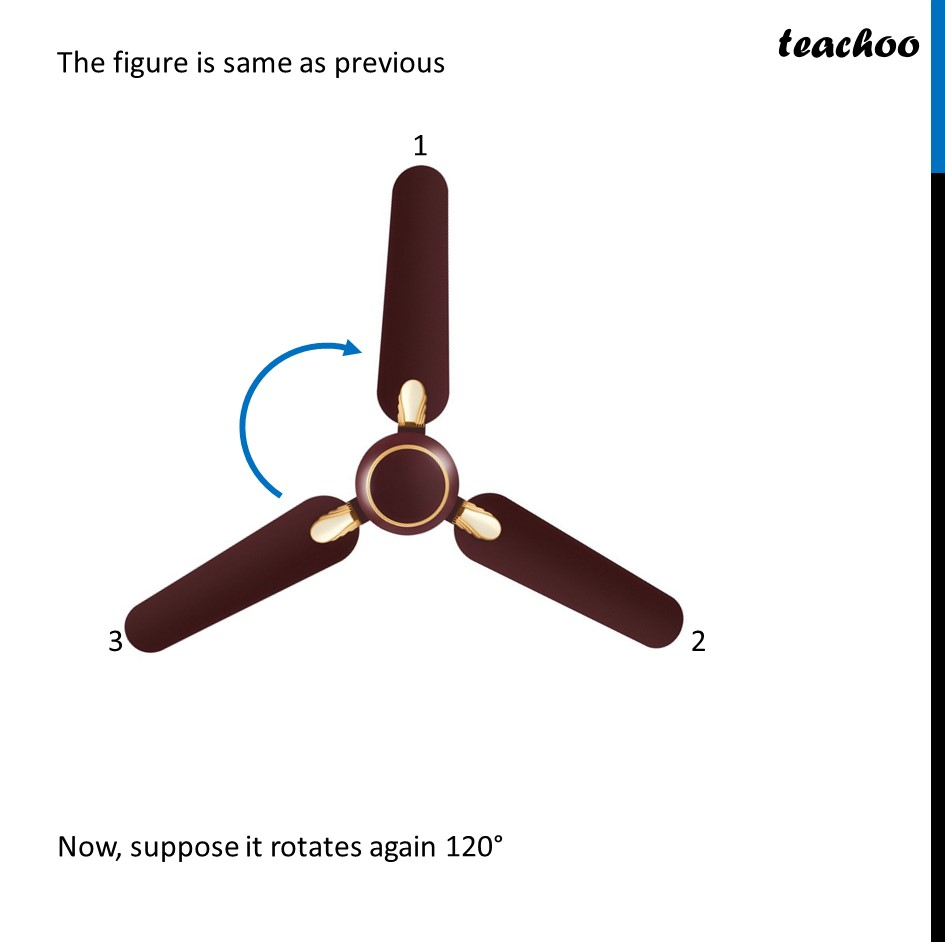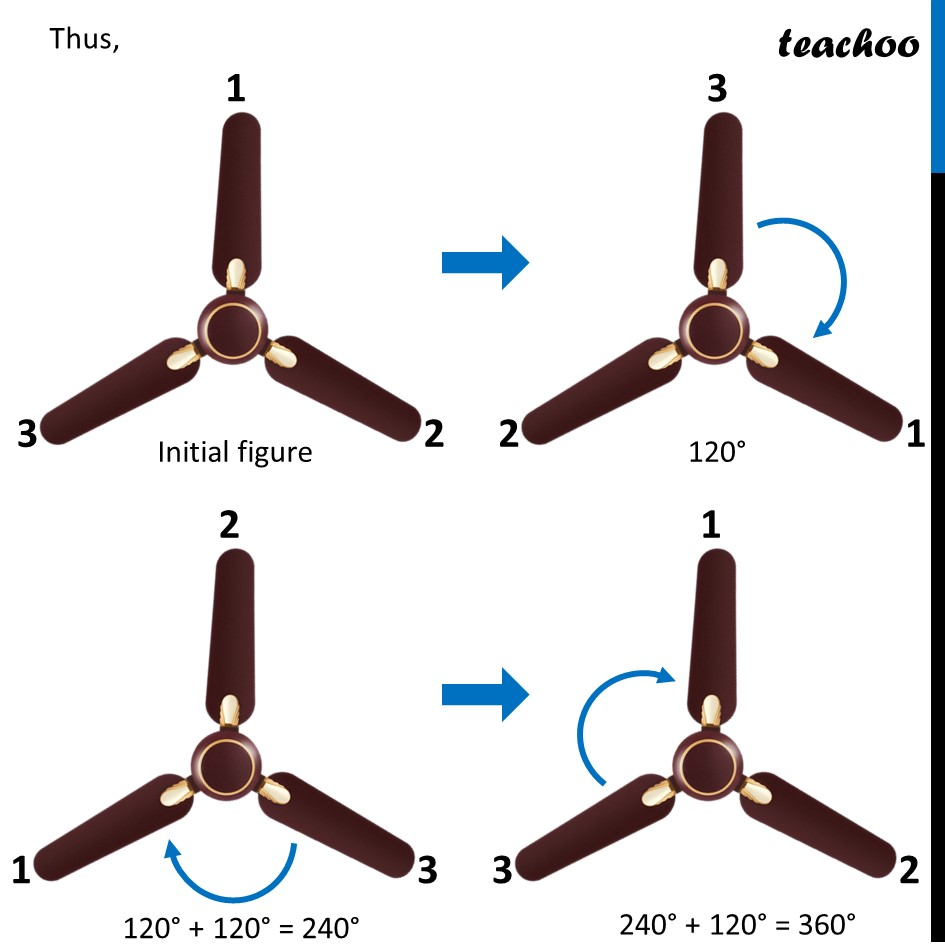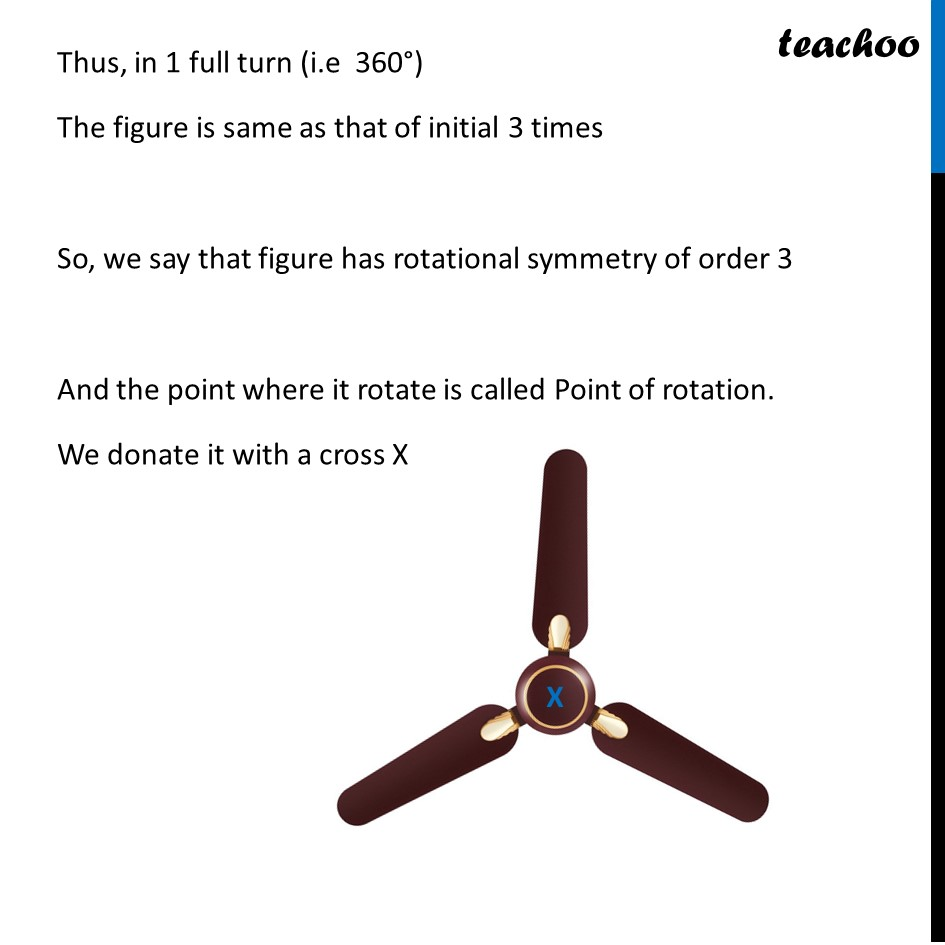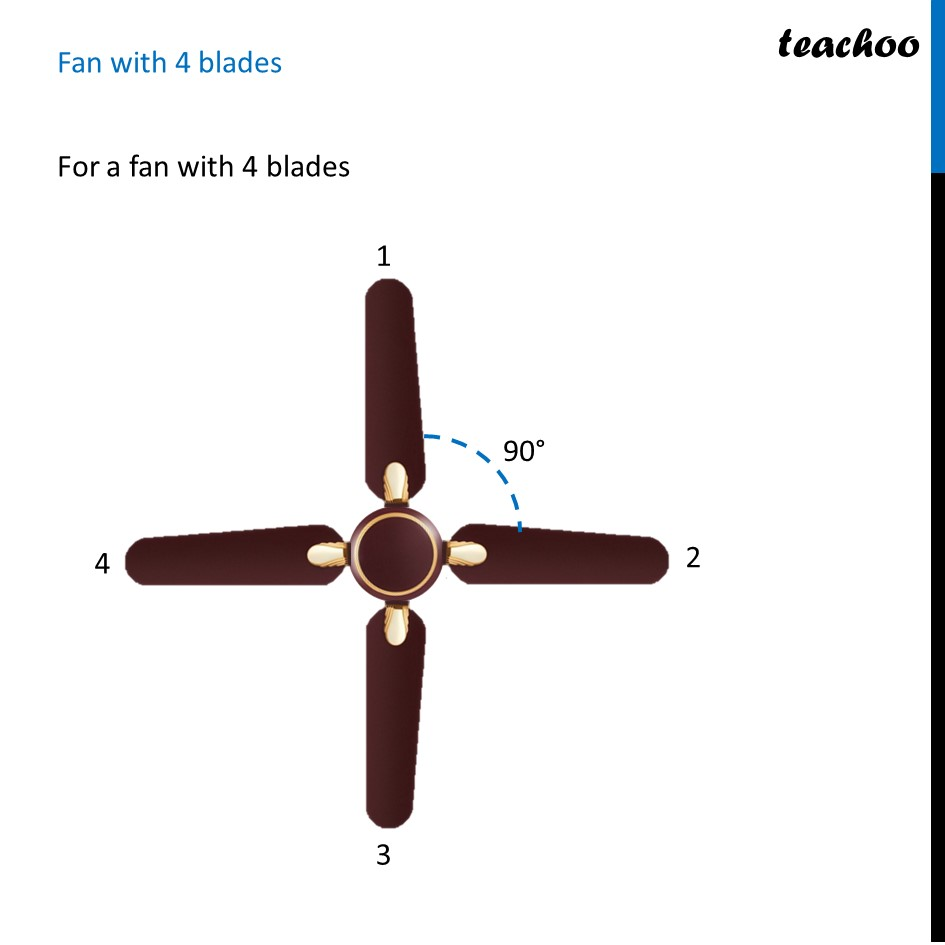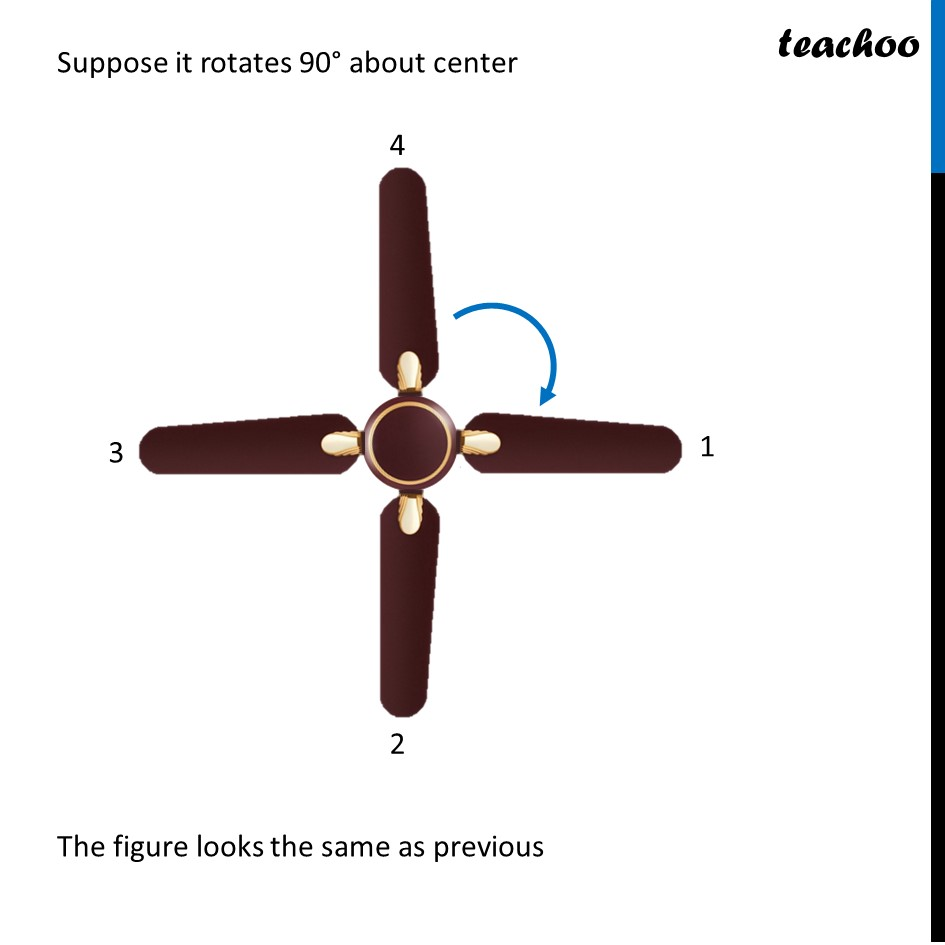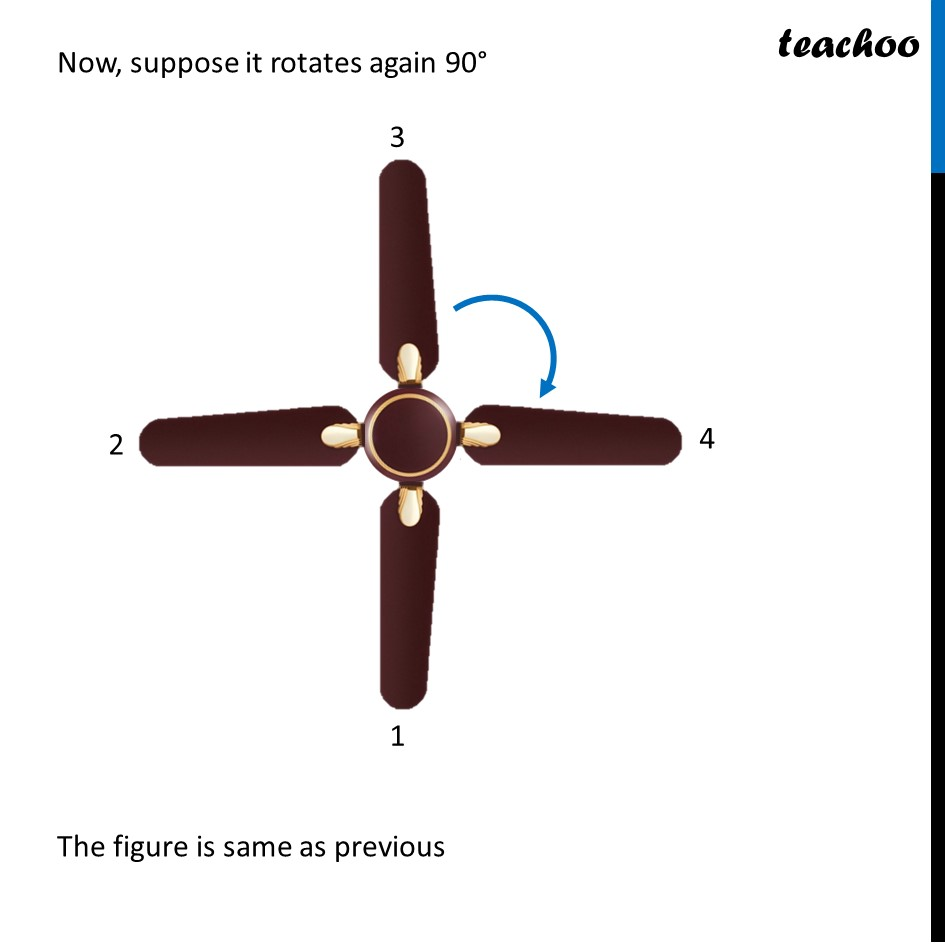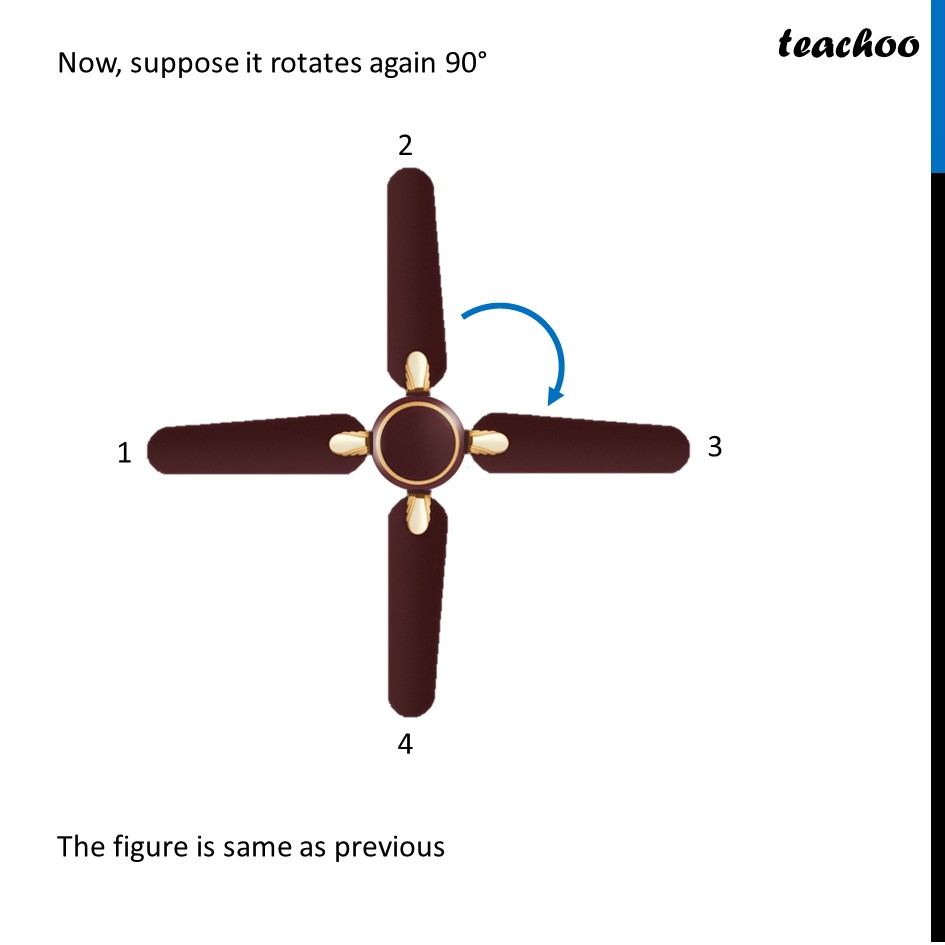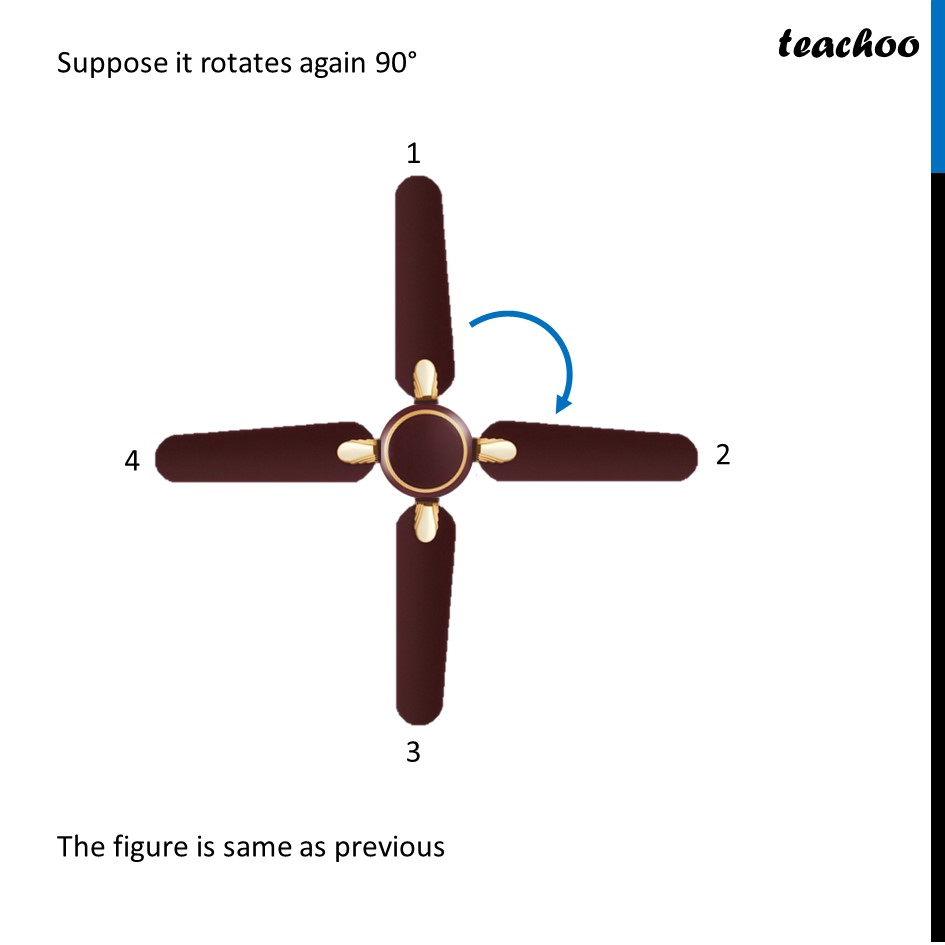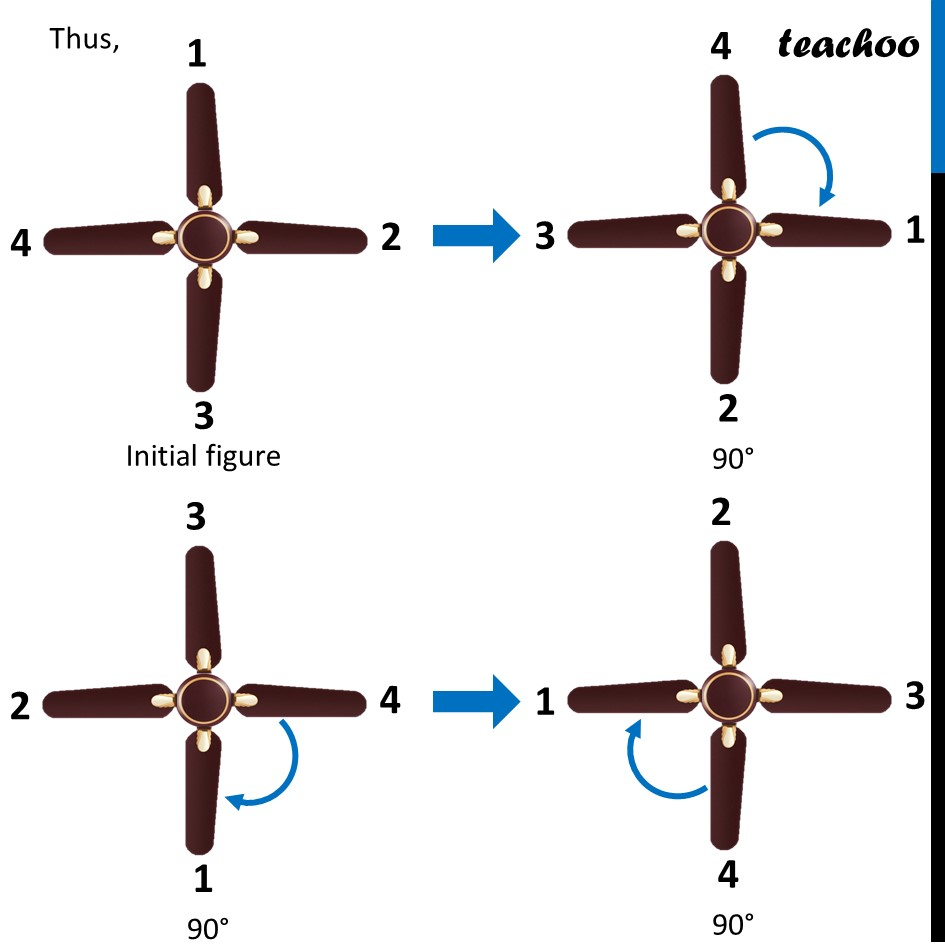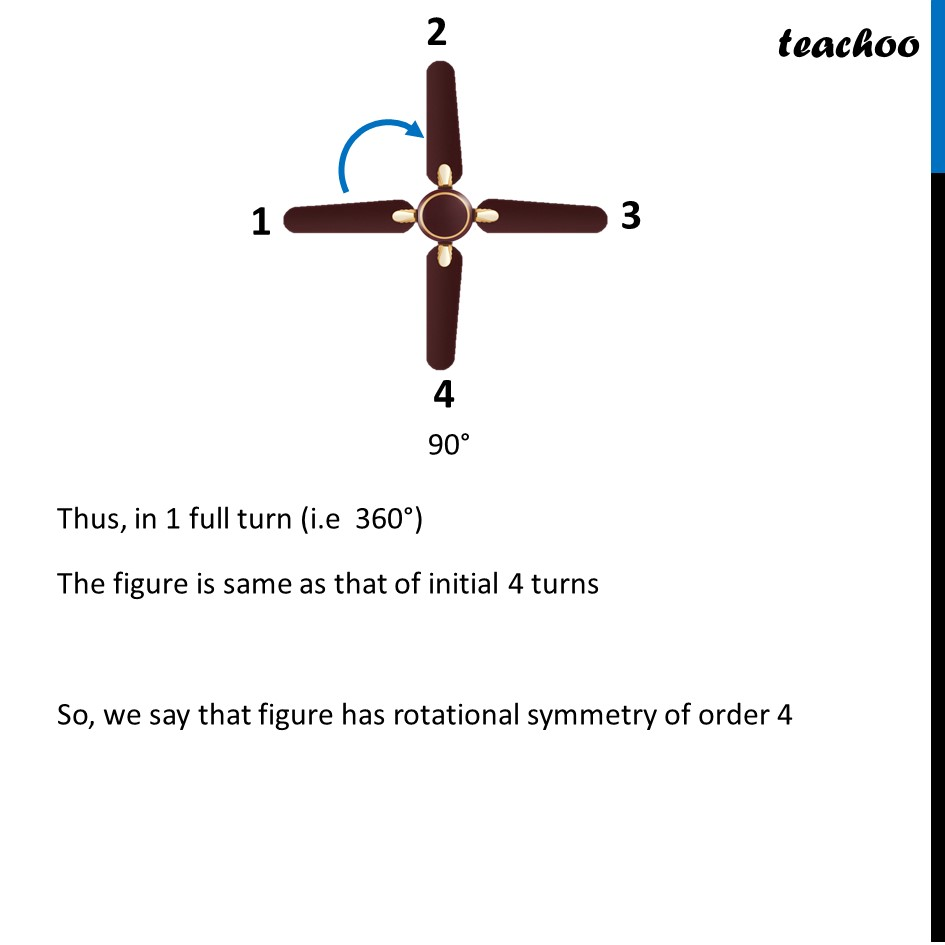Learn in your speed, with individual attention - Teachoo Maths 1-on-1 Class

### Transcript

Rotational symmetrySuppose we have a fan let’s rotate it around the center (marked as red cross) And suppose it rotate 120° The figure looks the same as previous Now, suppose it rotates again 120° The figure looks the same as previous The figure is same as previous Now, suppose it rotates again 120° Thus, 120° + 120° = 240° 240° + 120° = 360° Thus, in 1 full turn (i.e 360°) The figure is same as that of initial 3 times So, we say that figure has rotational symmetry of order 3 And the point where it rotate is called Point of rotation. We donate it with a cross X Fan with 4 bladesFor a fan with 4 blades Suppose it rotates 90° about center The figure looks the same as previous Now, suppose it rotates again 90° The figure is same as previous Now, suppose it rotates again 90° The figure is same as previous Suppose it rotates again 90° The figure is same as previous Thus, Initial figure Thus, in 1 full turn (i.e 360°) The figure is same as that of initial 4 turns So, we say that figure has rotational symmetry of order 4